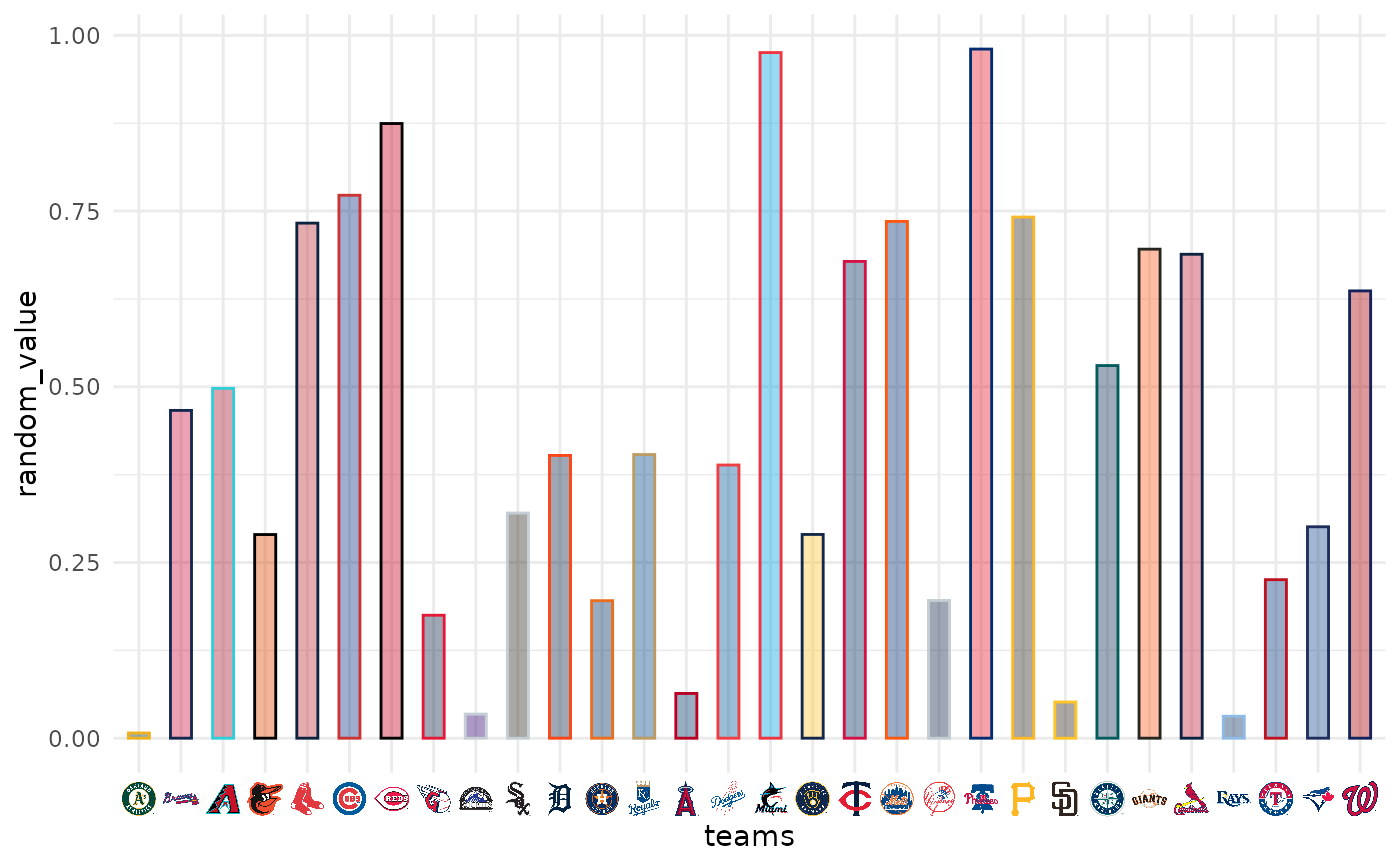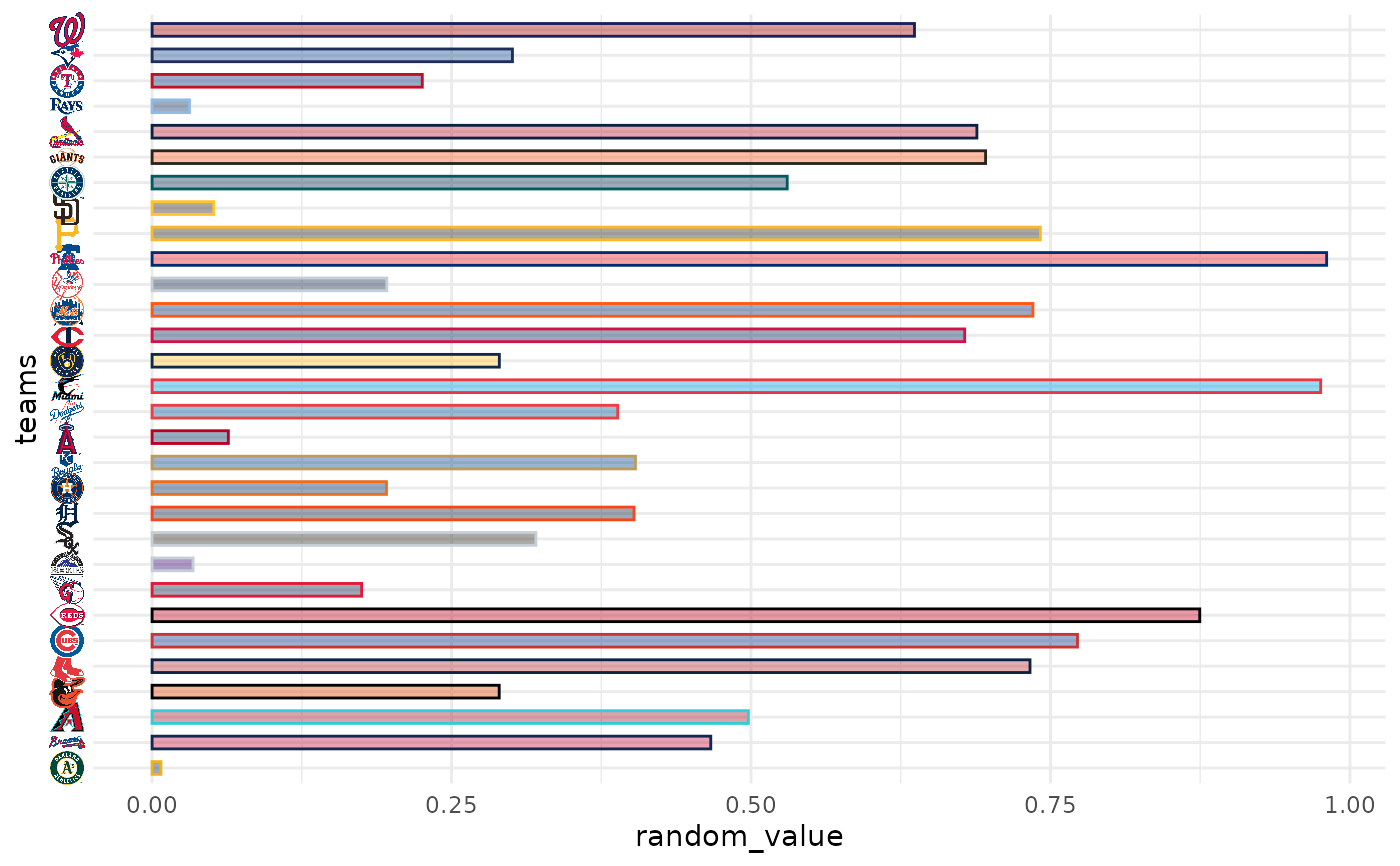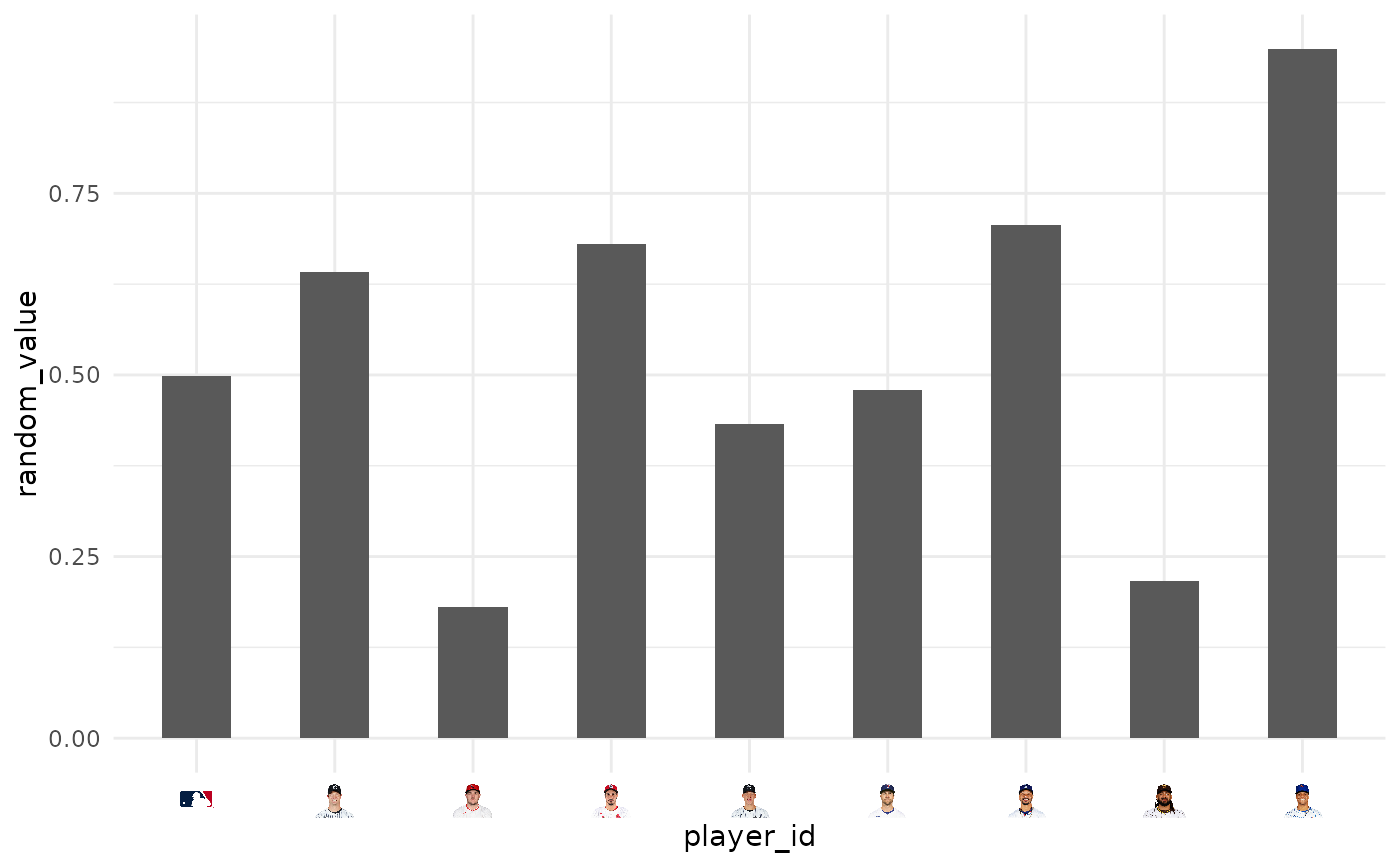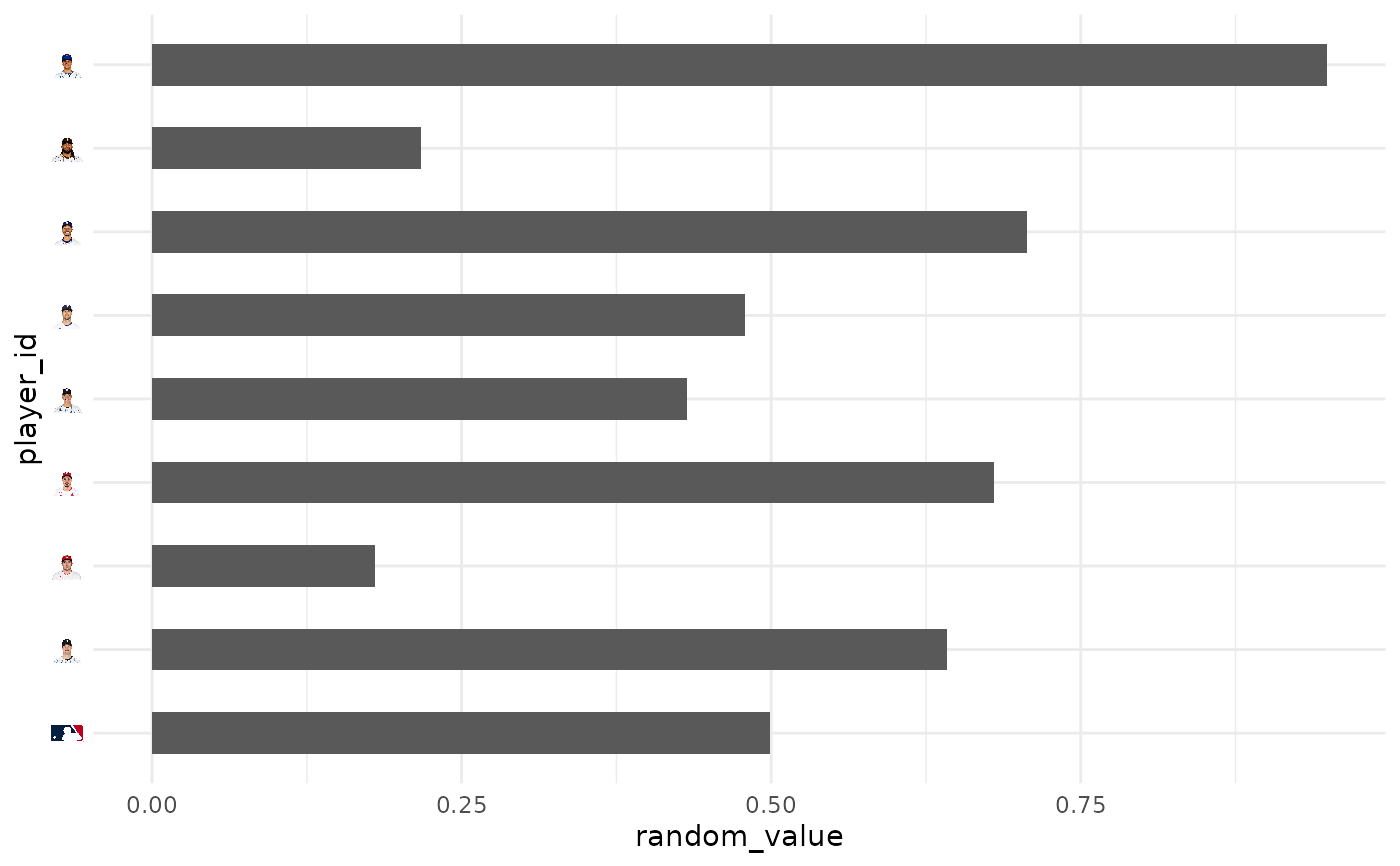In conjunction with the ggplot2::theme system, the following element_ functions enable images in non-data components of the plot, e.g. axis text.

• element_mlb_logo(), element_mlb_scoreboard_logo(), element_mlb_dot_logo(): draws MLB team logos instead of their abbreviations.

• element_mlb_dark_cap_logo() and element_mlb_light_cap_logo(): draws MLB team cap logos instead of their abbreviations.

• element_mlb_headshot(): draws MLB player headshots instead of their MLB IDs

• element_path(): draws images from valid image URLs instead of the URL.

## Usage

element_mlb_logo(
alpha = NULL,
colour = NA,
hjust = NULL,
vjust = NULL,
color = NULL,
size = 0.5
)

element_mlb_scoreboard_logo(
alpha = NULL,
colour = NA,
hjust = NULL,
vjust = NULL,
color = NULL,
size = 0.5
)

element_mlb_dot_logo(
alpha = NULL,
colour = NA,
hjust = NULL,
vjust = NULL,
color = NULL,
size = 0.5
)

element_mlb_dark_cap_logo(
alpha = NULL,
colour = NA,
hjust = NULL,
vjust = NULL,
color = NULL,
size = 0.5
)

element_mlb_light_cap_logo(
alpha = NULL,
colour = NA,
hjust = NULL,
vjust = NULL,
color = NULL,
size = 0.5
)

alpha = NULL,
colour = NA,
hjust = NULL,
vjust = NULL,
color = NULL,
size = 0.5
)

alpha = NULL,
colour = NA,
hjust = NULL,
vjust = NULL,
color = NULL,
size = 0.5
)

element_path(
alpha = NULL,
colour = NA,
hjust = NULL,
vjust = NULL,
color = NULL,
size = 0.5
)

## Arguments

alpha

The alpha channel, i.e. transparency level, as a numerical value between 0 and 1.

colour, color

The image will be colorized with this color. Use the special character "b/w" to set it to black and white. For more information on valid color names in ggplot2 see https://ggplot2.tidyverse.org/articles/ggplot2-specs.html?q=colour#colour-and-fill.

hjust, vjust

The horizontal and vertical adjustment respectively. Must be a numerical value between 0 and 1.

size

The output grob size in cm (!).

## Value

An S3 object of class element.

## Details

The elements translate MLB team abbreviations or MLB player IDs into logo images or headshots, respectively.

geom_mlb_logos(), geom_mlb_headshots(), and geom_from_path() for more information on valid team abbreviations, player ids, and other parameters.

## Examples

# \donttest{
library(mlbplotR)
library(ggplot2)

team_abbr <- valid_team_names()
# remove league logos from this example
team_abbr <- team_abbr[!team_abbr %in% c("AL", "NL", "MLB")]

df <- data.frame(
random_value = runif(length(team_abbr), 0, 1),
teams = team_abbr
)

# use logos for x-axis
ggplot(df, aes(x = teams, y = random_value)) +
geom_col(aes(color = teams, fill = teams), width = 0.5) +
scale_color_mlb(type = "secondary") +
scale_fill_mlb(alpha = 0.4) +
theme_minimal() +
theme(axis.text.x = element_mlb_logo())# use logos for y-axis
ggplot(df, aes(y = teams, x = random_value)) +
geom_col(aes(color = teams, fill = teams), width = 0.5) +
scale_color_mlb(type = "secondary") +
scale_fill_mlb(alpha = 0.4) +
theme_minimal() +
theme(axis.text.y = element_mlb_logo())#############################################################################
#############################################################################
library(mlbplotR)
library(ggplot2)

dfh <- data.frame(
random_value = runif(9, 0, 1),
player_id = c("594798",
"592450",
"605141",
"665742",
"545361",
"665487",
"571448",
"0",
"543037")
)

ggplot(dfh, aes(x = player_id, y = random_value)) +
geom_col(width = 0.5) +
theme_minimal() +